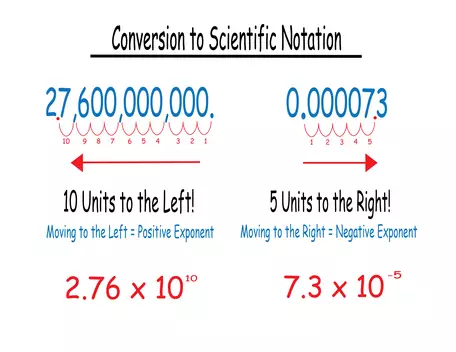# Scientific NotationManaging really big and really small numbers in the Earth sciences

## An introduction to scientific notation

The use of scientific notation is common across the natural sciences, and the geosciences are no exception. That's probably because it is an efficient way of dealing with very large or very small numbers - those with lots of zeros on either side of the decimal point - and the geosciences are replete with very large and very small numbers. The age of the Earth, for example, is 4.54 billion years or 4,540,000,000 years, but it is more compact to write it as 4.54 x 109 years. A carbon atom nucleus has a radius of 2.7 x 10-15 m or 0.0000000000000027 meters. It is no fun to have to write all those zeros especially when they are really just placeholders. Moreover, typing that many zeros into a calculator may not be possible and putting them into a spreadsheet is cumbersome and can lead to transcription errors.

### Key Terms

In 4.54 x 109 the number 4.54 is the coefficient. The coefficient can be any real number, positive or negative but generally we write coefficients only between 1 and 10.

The number 9 is the exponent. In scientific notation the exponent will always be an integer but may be positive or negative. The number 10 is called the base.

### Decoding scientific notation: What does 4.54 x 109 mean?

We take the number 4.54 and multiply it by 10 raised to the 9th power. This translates to 4.54 x 10 x 10 x 10 x 10 x 10 x 10 x 10 x 10 x 10. And what about 2.7 x 10-15? That is 2.7 times 10 raised to the negative 15th power. Recall raising a number to the negative power is the same as dividing by that number, so 10-1 is 1/10 or 0.1. So 2.7 x 10-15 becomes 2.7 x 0.1 x 0.1 x 0.1 x 0.1 x 0.1 x 0.1 x 0.1 x 0.1 x 0.1 x 0.1 x 0.1 x 0.1 x 0.1 x 0.1 x 0.1. Talk about cumbersome!

## How do I use scientific notation?

### To convert a number in standard format to scientific notation:× Conversion to Scientific NotationLet's say you want to express the speed of light (300,000,000 meters per second) in scientific notation.

Step 1 - Determine if the number is "large" or "small".

Step 2 - Determine which way to move the decimal.

Step 3 - Determine the number of places you need to move the decimal until the first number is greater than 1 but less than 10.

Step 4 - Determine the sign of the exponent as negative or positive.

Step 5 - Combine steps 3 and 4 to write the exponent.

Step 6 - Lastly, write the coefficient x 10exponent. Be sure to include units!

You are given the hydraulic conductivity of a sandstone as 0.00005 centimeters per second (cm/s). How would you write this in scientific notation? Hydraulic conductivity measures how easily fluid can flow through porous media (e.g. sediment or rocks). These rates are extremely low and are part of the reason it is hard to clean up contaminated groundwater.

Step 1 - Determine if the number is "large" or "small".

Step 2 - Determine which way to move the decimal.

Step 3 - Determine the number of places you need to move the decimal until the first number is greater than 1 but less than 10.

Step 4 - Determine the sign of the exponent as negative or positive.

Step 5 - Combine steps 3 and 4 to write the exponent.

Step 6 - Lastly write the coefficient x 10exponent. Be sure to include units!

### To convert a number in scientific notation into standard decimal form:

You essentially reverse the process described above.

You are given the concentration of methane in the atmosphere as 1.895 x 10-6 milligrams per liter (mg/L) and asked to express this in standard decimal format. Methane, like carbon dioxide, is an important greenhouse gas.

Step 1 - Determine if the exponent is positive or negative. If the exponent is positive, you will move the decimal to the right if negative then to the left.

Step 2 - Determine the number of places to move the decimal by looking at the magnitude of the exponent.

Step 3 - Write the coefficient using zeros as placeholders between the coefficient and the decimal. As always don't forget your units!

### Scientific notation on calculators and spreadsheets

There are other ways you may see scientific notation represented. For example, on some calculators and spreadsheets you might see 3e8 instead of 3 x 108 others might use E, EE, EX, or EXP. Alternatively some may use a "carrot" ^ symbol to indicate the exponent (3 x 10^8). On some calculators you need to select the SCI mode in order to display in scientific notation. The good thing is that these are all just different ways of representing the same number and many of the calculator companies have online tutorials if you need more help, including TI, Sharp, and HP.

## Where do you use scientific notation in Earth science?

The are many examples, but anywhere you are dealing with very large or very small numbers, scientific notation is recommended. For example:

• Determining rates of sea floor spreading based on oceanic crust age.
• Determining the volume of material erupted by volcanoes.
• Calculating residence times and fluxes for various elements in biogeochemical cycles
• e.g., nutrient cycles, carbon cycle, hydrologic cycle
• Determining average watershed denudation rate based on sediment transport budgets.
• Wavelength analysis of X-ray diffraction data
• Estimating aquifer yields
• Comparing rates of weathering in different climates